## UGC NET CS 2017 Nov- paper-2

 Question 1
If the time is now 4 O’clock, what will be the time after 101 hours from now ?
 A 9 O’Clock B 8 O'Clock C 5 O’Clock D 4 O’Clock
genereal-aptitude       Time-clock
Question 1 Explanation:
Per day 24 hours.
Present time given 4 O’clock.
Method-1: After 24+24+24+24=96. it means after 96 hours again time is 4 O’clock.
Remaining 5 hours. So, total 4+5=9 O’Clock
Method-2: Perform modulus operation by 24. So, 101%24=5
= Given time + modules time
= 4+5=9 O’Clock
 Question 2Find the boolean product A⊙B of the two matrices.
 ABCDEngineering-Mathematics       Linear-Algebra
Question 2 Explanation:
In this problem, they given boolean product A⊙B of two matrices.
The boolean product truth table isAccording to the truth table, we have to perform matrix multiplication.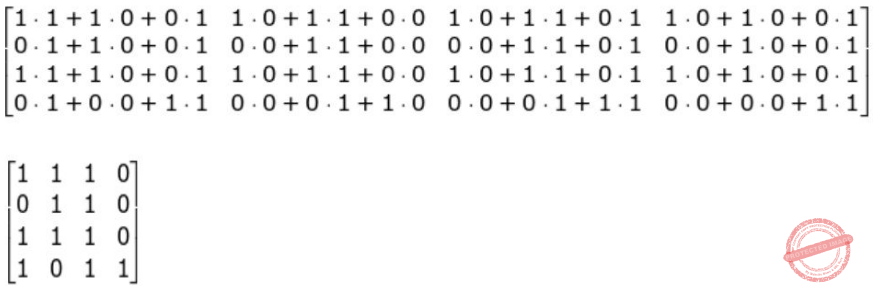Question 3
How many distinguishable permutations of the letters in the word BANANA are there ?
 A 720 B 120 C 60 D 360
Engineering-Mathematics       Probability
Question 3 Explanation:
Total distinguishable permutations means no repetition.
BANANA= 6 letters
B is appearing 1 time
A is appearing 3 times
N is appearing 2 times
So, = 6! / (3! * 2!)
= 60
 Question 4
The Octal equivalent of the binary number 1011101011 is :
 A 7353 B 1353 C 5651 D 5657
Digital-Logic-Design       Number-Systems
Question 4 Explanation:
We have to divide binary number into 3 bit pairs from LSB.
1 011 101 011
1 3 5 3
(1011101011)​ 2​ = (1353)​ 8
 Question 5
Let P and Q be two propositions, ¬ (P ↔ Q) is equivalent to:
(I) P ↔ ¬ Q
(II) ¬ P ↔ Q
(III) ¬ P ↔ ¬ Q
(IV) Q → P
 A Only (I) and (II) B Only (II) and (III) C Only (III) and (IV) D None of the above
Engineering-Mathematics       Propositional-Logic
Question 5 Explanation: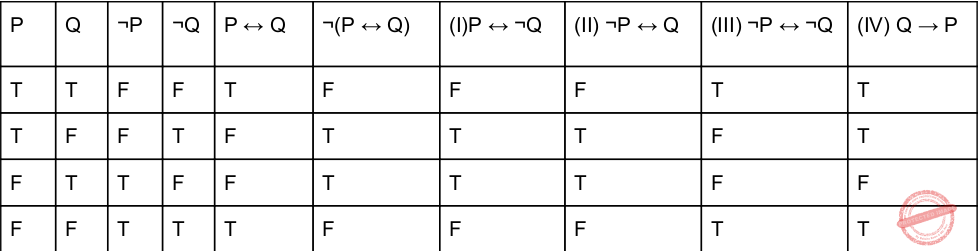So, (I) and (Ii) are TRUE.
 Question 6
‘ptrdata’ is a pointer to a data type. The expression *ptrdata++ is evaluated as (in C++) :
 A *(ptrdata++) B (*ptrdata)++ C * (ptrdata) ++ D Depends on compiler
Programming-in-c++       Data-types
Question 6 Explanation:
Normally ++ having higher precedence than * but both are unary operators in C++.
So, the precedence ptrdata++ will evaluate first. So, we are given parenthesis (ptrdata++).
Second precedence is * and finally *(ptrdata++)
 Question 7
The associativity of which of the following operators is Left to Right, in C++ ?
 A Unary Operator B Logical not C Array element access D addressof
Programming-in-c++       Operators
Question 7 Explanation:Note: The array element access evaluates from left to right and starts index 0.
 Question 8
A member function can always access the data in __________ , (in C++).
 A the class of which it is member B the object of which it is member C the public part of its class D the private part of its class
Programming-in-c++       Functions
Question 8 Explanation:
→ A member function can always access the data in the class of which it is member.
→ Functions within classes can access and modify (unless the function is constant) data members without declaring them, because the data members are already declared in the class.
 Question 9
Which of the following is not correct for virtual function in C++ ?
 A Must be declared in public section of class B Virtual function can be static C Virtual function should be accessed using pointers. D Virtual function is defined in base class
Programming-in-c++       Virtual-Functions
Question 9 Explanation:
→ Virtual functions are called only for objects of class types, you cannot declare global or static functions as virtual.
 Question 10
Which of the following is not correct (in C++) ?
 A Class templates and function templates are instantiated in the same way B Class templates differ from function templates in the way they are initiated C Class template is initiated by defining an object using the template argument D Class templates are generally used for storage classes E None of the above
Programming-in-c++       Functions
Question 10 Explanation:
(1) TRUE: Class templates and function templates are instantiated in the same way
(2) FALSE: Class templates differ from function templates in the way they are initiated
(3) FALSE: Class template is initiated by defining an object using the template argument
(4)FALSE: Class templates are generally used for storage classes
 Question 11
Which of the following is/are true with reference to ‘view’ in DBMS ?
(a) A ‘view’ is a special stored procedure executed when certain event occurs.
(b) A ‘view’ is a virtual table, which occurs after executing a pre-compiled query. code:
 A Only (a) is true B Only (b) is true C Both (a) and (b) are true D Neither (a) nor (b) are true
Database-Management-System       SQL
Question 11 Explanation:
VIEW is a virtual table based on the result set of a SQL statement.
→ In SQL, a view is a virtual table based on the result-set of an SQL statement.
→ A view contains rows and columns, just like a real table. The fields in a view are fields from one or more real tables in the database.
→ You can add SQL functions, WHERE, and JOIN statements to a view and present the data as if the data were coming from one single table.
CREATE VIEW Syntax:
CREATE VIEW view_name AS
SELECT column1, column2, ...
FROM table_name
WHERE condition;
Note: A view always shows up-to-date data! The database engine recreates the data, using the view's SQL statement, every time a user queries a view.
 Question 12
In SQL, __________ is an Aggregate function.
 A SELECT B CREATE C AVG D MODIFY
Database-Management-System       SQL
Question 12 Explanation:
Aggregate function is AVG. The AVG() function returns the average value of a numeric column.
 Question 13
Match the following with respect to RDBMS :A (a)-(iii), (b)-(iv), (c)-(i), (d)-(ii) B (a)-(iv), (b)-(iii), (c)-(ii), (d)-(i) C (a)-(iv), (b)-(ii), (c)-(iii), (d)-(i) D (a)-(ii), (b)-(iii), (c)-(iv), (d)-(i)
Database-Management-System       Relational-databases
Question 13 Explanation:
→ Entity Integrity won’t support duplicate rows in a table
→ Domain Integrity enforces valid entries for a column
→ Referential integrity rows can’t be deleted which are used by other records
→ User defined integrity enforces some specific business rule that do not fall into entity or domain
 Question 14
In RDBMS, different classes of relations are created using __________ technique to prevent modification anomalies.
 A Functional Dependencies B Data integrity C Referential integrity D Normal forms
Database-Management-System       Relational-databases
Question 14 Explanation:
Normal forms in order to reduce data redundancy and improve data integrity. Normal forms to prevent modification anomalies.
Example anomalies:
1. Insertion anomaly
2. Deletion and Updation anomaly
 Question 15
__________ SQL command changes one or more fields in a record.
 A LOOK-UP B INSERT C MODIFY D CHANGE
Database-Management-System       SQL
Question 15 Explanation:
The ALTER TABLE statement is used to add, delete, or modify columns in an existing table.
The ALTER TABLE statement is also used to add and drop various constraints on an existing table.
Syntax:ALTER TABLE table_name MODIFY COLUMN column_name datatype;
 Question 16
Consider an array representation of an n element binary heap where the elements are stored from index 1 to index n of the array. For the element stored at index i of the array (i<=n), the index of the parent is:
 A floor ((i+1)/2) B ceiling ((i+1)/2) C floor (i/2) D ceiling (i/2)
Algorithms       Sorting
Question 16 Explanation:
Parent Node is at index: ⌊i/2⌋
Left Child is at index: 2i
Right child is at index: 2*i+1
 Question 17
The following numbers are inserted into an empty binary search tree in the given order: 10, 1, 3, 5, 15, 12, 16 What is the height of the binary search tree ?
 A 3 B 4 C 5 D 6
Algorithms       Searching
Question 17 Explanation:Height of the binary search tree = 3
 Question 18
Let G be an undirected connected graph with distinct edge weight. Let Emax be the edge with maximum weight and E​ min​ the edge with minimum weight. Which of the following statements is false?
 A Every minimum spanning tree of G must contain E​ min. B If E​ max​ is in minimum spanning tree, then its removal must disconnect G. C No minimum spanning tree contains E​ max. D G has a unique minimum spanning tree.
Algorithms       Minimum-Spanning-Tree
Question 18 Explanation:Minimum Spanning Tree: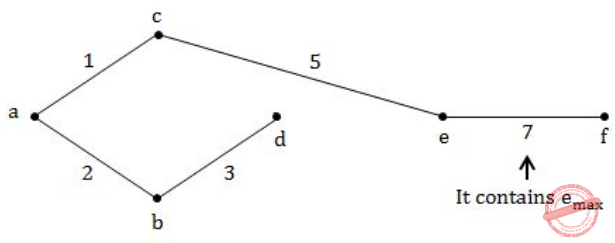Question 19
A list of n strings, each of length n, is sorted into lexicographic order using merge – sort algorithm. The worst case running time of this computation is :
 A O(n log n) B O(n​ 2​ log n) C O(n​ 2​ + log n) D O(n​ 3​ )
Algorithms       Sorting
Question 19 Explanation:
1.The comparison is strictly follow the Dictionary based order.
2.The length of the string is n, the time taken is to be O(n) for each comparison.
3. For level -1(bottom-up order): Every element has to be compared with other element the number of comparisons is O(n/2).
4. For level -1(bottom-up order): Total time taken by one level is O(n​ 2​ ).
5. For copying level to level the time taken by O(n​ 2​ ).
So, For level -1= O(n​ 2​ )+ O(n​ 2​ )
6. Second level O(n​ 2​ )+ O(n​ 2​ ).
;
;
;
Final n level (logn)*O(n​ 2​ ) = O(n​ 2​ logn)
 Question 20
Which of the following devices takes data sent from one network device and forwards it to the destination node based on MAC address ?
 A Hub B Modem C Switch D Gateway
Computer-Networks
Question 20 Explanation:
→ Switch takes data sent from one network device and forwards it to the destination node based on MAC address.
→ Physical layer uses HUB and repeater
→ Data link layer uses Switch. MAC address comes into data link layer.
 Question 21
__________ do not take their decisions on measurements or estimates of the current traffic and topology.
 A Static algorithms B Adaptive algorithms C Non - adaptive algorithms D Recursive algorithms
Computer-Networks       Topologies
Question 21 Explanation:
Non-Adaptive Routing Algorithm: These algorithms do not base their routing decisions on measurements and estimates of the current traffic and topology. Instead the route to be taken in going from one node to the other is computed in advance, off-line, and downloaded to the routers when the network is booted. This is also known as static routing. This can be further classified as:
1. Flooding
2. Random Walk
 Question 22
The number of bits used for addressing in Gigabit Ethernet is __________.
 A 32 bit B 48 bit C 64 bit D 128 bit
Computer-Networks       Ethernet
Question 22 Explanation:
→ The number of bits used for addressing in Gigabit Ethernet is 48 bit
→ The Ethernet frame is the packet that delivers data between stations.
→ Each frame is made up of bits which are organized into different fields. The four main fields are the destination Ethernet address field, source Ethernet address field, data field and frame check sequence field.
 Question 23
Which of the following layer of OSI Reference model is also called end-to-end layer?
 A Network layer B Data layer C Session layer D Transport layer
Computer-Networks       OSI-TCP-layers
Question 23 Explanation:
→ Data link layer-Process to Process connectivity
→ Network layer-Host to Host connectivity
→ Transport layer-End to End connectivity
→ Presentation layer-Encryption and Decryption
 Question 24
The IP address __________ is used by hosts when they are being booted
 A 0.0.0.0 B 1.0.0.0 C 1.1.1.1 D 255.255.255.255
Computer-Networks       OSI-TCP-layers
Question 24 Explanation:
We can also call default route.
If a packet destination address is not known, then if the default route is not known, then if the default route is present in the routing table of the router, then the packet is not discarded but forwarded to the next router. 0.0.0.0
 Question 25
Which of the following regular expressions, each describing a language of binary numbers (MSB to LSB) that represents non negative decimal values, does not include even values ?
 A 0*1​ +​ 0*1* B 0*1*0​ +​ 1* C 0*1*0*1​ + D 0+1*0*1*
Theory-of-Computation       Regular-Expression
Question 25 Explanation:
Binary numbers can easily find for odd numbers using LSB is 1 and even numbers LSB is 0. Option C won’t contain even numbers
 Question 26
Match the description of several parts of a classic optimizing compiler in List - I, with the names of those parts in List - II:A (a)-(iii), (b)-(iv), (c)-(ii), (d)-(i) B (a)-(iv), (b)-(iii), (c)-(ii), (d)-(i) C (a)-(ii), (b)-(iv), (c)-(i), (d)-(iii) D (a)-(ii), (b)-(iv), (c)-(iii), (d)-(i)
Compiler-Design       Compilers
Question 26 Explanation:
Parser→ A part of a compiler that is responsible for recognizing synta
Scanner→ An IR-to-IR transformer that tries to improve the IR program in some way (Intermediate representation)
Semantic Analysis→ A part of a compiler that understand the meaning of variable names and other symbols and checks that they are used in ways consistent with their definitions
Optimizer→ An IR-to-IR transformer that tries to improve the IR program in some way (Intermediate representation)
 Question 27
In Distributed system, the capacity of a system to adapt the increased service load is called __________ .
 A Tolerance B Scalability C Capability D Loading
Compiler-Design       Linker-Loader
Question 27 Explanation:
→ The capacity of a system to adapt the increased service load is called scalability.
→ Scalability is the capability of a system, network, or process to handle a growing amount of work, or its potential to be enlarged to accommodate that growth.
Note: A scalable system is any system that is flexible with its number of components.
 Question 28
In __________ disk scheduling algorithm, the disk head moves from one end to other end of the disk, serving the requests along the way. When the head reaches the other end, it immediately returns to the beginning of the disk without serving any requests on the return trip.
 A LOOK B SCAN C C-LOOK D C-SCAN
Operating-Systems       Disk-Scheduling
Question 28 Explanation:
Circular scanning works just like the elevator to some extent. It begins its scan toward the nearest end and works it way all the way to the end of the system. Once it hits the bottom or top it jumps to the other end and moves in the same direction. Keep in mind that the huge jump doesn't count as a head movement.
 Question 29
Suppose there are six files F1, F2, F3, F4, F5, F6 with corresponding sizes 150 KB, 225KB,75 KB, 60 KB, 275 KB and 65 KB respectively. The files are to be stored on a sequential device in such a way that optimizes access time. In what order should the files be stored ?
 A F5, F2, F1, F3, F6, F4 B F4, F6, F3, F1, F2, F5 C F1, F2, F3, F4, F5, F6 D F6, F5, F4, F3, F2, F1
Operating-Systems       Page-Replacement-algorithm
Question 29 Explanation:
Here, clearly mentioned that files are stored in sequential device. It is like optimal merge pattern. We want to get optimum access time, first sort all the files according to their sizes. For sorting it will take worst case O(nlogn) but will give optimal result. So, Option D is correct order.
Note: Merging files required m+n-1 comprisions
 Question 30
Which module gives control of the CPU to the process selected by the short - term scheduler ?
 A Dispatcher B Interrupt C Schedular D Threading
Operating-Systems       Process-Threads
Question 30 Explanation:
The CPU-scheduling function is the dispatcher, which is the module that gives control of the CPU to the process selected by the short-term scheduler. It receives control in kernel mode as the result of an interrupt or system call. The functions of a dispatcher involve the following:
1. Context switches, in which the dispatcher saves the state (also known as context) of the process or thread that was previously running; the dispatcher then loads the initial or previously saved state of the new process.
2. Switching to user mode.
3. Jumping to the proper location in the user program to restart that program indicated by its new state.
The dispatcher should be as fast as possible, since it is invoked during every process switch. During the context switches, the processor is virtually idle for a fraction of time, thus unnecessary context switches should be avoided. The time it takes for the dispatcher to stop one process and start another is known as the dispatch latency.
 Question 31
Two atomic operations permissible on Semaphores are __________ and __________.
 A wait, stop B wait, hold C hold, signal D wait, signal
Operating-Systems       Semaphores
Question 31 Explanation:
A semaphore is a variable or abstract data type used to control access to a common resource by multiple processes in a concurrent system such as a multitasking operating system. Two atomic operations are
1. wait: If the value of semaphore variable is not negative, decrement it by 1. If the semaphore variable is now negative, the process executing wait is blocked (i.e., added to the semaphore's queue) until the value is greater or equal to 1. Otherwise, the process continues execution, having used a unit of the resource.
2. signal: Increments the value of semaphore variable by 1. After the increment, if the pre-increment value was negative (meaning there are processes waiting for a resource), it transfers a blocked process from the semaphore's waiting queue to the ready queue.
 Question 32
Software does not wear-out in the traditional sense of the term, but software does tend to deteriorate as it evolves, because :
 A Software suffers from exposure to hostile environments. B Defects are more likely to arise after software has been used often. C Multiple change requests introduce errors in component interactions. D Software spare parts become harder to order.
Software-Engineering       Software-configuration-management
Question 32 Explanation:
Software is not susceptible to the same environmental problems that cause hardware to wear out. In theory, therefore, the failure rate curve for software should take the form shown below.Question 33
Software re-engineering is concerned with:
 A Re-constructing the original source code from the existing machine (low level) code program and modifying it to make it more user - friendly. B Scrapping the source code of a software and re writing it entirely from scratch. C Re-organising and modifying existing software systems to make them more maintainable. D Translating source code of an existing software to a new machine (low level) language.
Software-Engineering       Software-Reengineering
Question 33 Explanation:
Software re-engineering is concerned with Re-organising and modifying existing software systems to make them more maintainable.
→ Reengineering is the modification of a software system that takes place after it has been reverse engineered, generally to add new functionality, or to correct errors.
 Question 34
Which of the following is not a key issue stressed by an agile philosophy of software engineering ?
 A The importance of self-organizing teams as well as communication and collaboration between team members and customers. B Recognition that change represents opportunity. C Emphasis on rapid delivery of software that satisfies the customer. D Having a separate testing phase after a build phase.
Software-Engineering       Software-process-models
Question 34 Explanation:
→ Agile software development testing is completed in the same iteration as programming. One phase is completed in its entirety before moving on to the next phase because testing is done in every iteration which develops a small piece of the software users can frequently use those new pieces of software and validate the value.
 Question 35
What is the normal order of activities in which traditional software testing is organized?
(a) Integration Testing
(b) System Testing
(c) Unit Testing
(d) Validation Testing
 A (c),(a),(b),(d) B (c),(a),(d),(b) C (d),(c),(b),(a) D (b),(d),(a),(c)
Software-Engineering       Software-testing
Question 35 Explanation:Unit testing:​ Unit testing starts at the centre and each unit is implemented in source code.
Integration testing:​ An integration testing focuses on the construction and design of the software.
Validation testing:​ Check all the requirements like functional, behavioral and performance requirement are validate against the construction software.
System testing:​ System testing confirms all system elements and performance are tested entirely.
 Question 36
Which of the following testing techniques ensures that the software product runs correctly after the changes during maintenance ?
 A Path Testing B Integration Testing C Unit Testing D Regression Testing
Software-Engineering       Software-testing
Question 36 Explanation:
→ Regression testing is the re-execution of some subset of tests that have already been conducted to ensure that changes have not propagated unintended side effects Whenever software is corrected, some aspect of the software configuration (the program, its documentation, or the data that support it) is changed.
→ Regression testing helps to ensure that changes (due to testing or for other reasons) do not introduce unintended behavior or additional errors.
→ Regression testing may be conducted manually, by re-executing a subset of all test cases or using automated capture/playback tools.
 Question 37
Which of the following Supercomputers is the fastest Supercomputer ?
 A Sunway TaihuLight B Titan C Piz Daint D Sequoia
Computer-Organization       Super-computers
Question 37 Explanation:
→ The Sunway TaihuLight is a Chinese supercomputer which, as of November 2018, is ranked third in the TOP500 list, with a LINPACK benchmark rating of 93 petaflops.[ The name is translated as divine power, the light of Taihu Lake.
 Question 38
Which of the following statements about ERP system is true ?
 A Most ERP software implementations fully achieve seamless integration B ERP software packages are themselves combinations of separate applications for manufacturing, materials, resource planning, general ledger, human resources, procurement and order entry. C Integration of ERP systems can be achieved in only one way D An ERP package implemented uniformly throughout an enterprise is likely to contain very flexible connections to allow charges and software variations.
Software-Engineering       ERP
Question 38 Explanation:
ERP software packages are themselves combinations of separate applications for manufacturing, materials, resource planning, general ledger, human resources, procurement and order entry.
 Question 39
Which of the following is not a Clustering method ?
 A K-Mean method B Self Organizing feature map method C K-nearest neighbor method D Agglomerative method
Database-Management-System       Data-warehouse-and-data-mining
Question 39 Explanation:Question 40
Which of the given wireless technologies used in IoT, consumes the least amount of power ?
 A Zigbee B Bluetooth C Wi-Fi D GSM/CDMA
Computer-Networks       OSI-TCP-layers
Question 40 Explanation:
Bluetooth wireless technologies used in IoT, consumes the least amount of power
 Question 41
Which speed up could be achieved according to Amdahl’s Law for infinite number of processes if 5% of a program is sequential and the remaining part is ideally parallel ?
 A Infinite B 5 C 20 D 50
Computer-Networks       Amdahl’s-Law
Question 41 Explanation:
Step-1: Speed up for infinite number of process uses Amdahl's law formula is
S = 1 / (1-P)
where,
P is parallel part of program,
sequential part of program is 5%
. = 1 - sequential part
= 1 - 0.05 (or 5%)
= 0.95 (or 95%)
Step-2: Apply into the formula is
S = 1 / (1-P)
S = 1 / (1-0.95)
S = 1 / 0.05
S = 20
 Question 42
Negation of the proposition ∃x H(x) is:
 A ∃x ⌐H(x) B ∀x ⌐H(x) C ∀x H(x) D ⌐x H(x)
Engineering-Mathematics       Propositional-Logic
Question 42 Explanation:
∃x H(x) = ∀x ⌐H(x)
 Question 43
The output of the following combinational circuit is F.A P1+P’2P3 B P1+P’2P’3 C P​ 1​ +P​ 2​ P’​ 3 D P​’ 1​ +P​ 2​ P​ 3
Digital-Logic-Design       Combinational-Circuits
Question 43 Explanation:
Method1: Simplification by expansion.
F= (P1+P'2+P'3 )( P1+ P'2+ P3 )( P1+P2+P'3) ← POS
=(P1+ P1P'2 +P1P3 + P'2P1 + P'2 P'2 + P'2P3 + P'3P1+ P'3P'2+P'3P3 )(P1+P2+P'3)
=(P1(1+P'2+P3 + P'2 + P'3)+P'2 (1+P3+P'3) + 0)(P1+P2+P'3 =(P1+P'2)(P1+P2+P'3)
=P1(1+P2+P'3+P'2)+P'2P2+P'2P'3
=P1 + 0 + P'2P'3
=P1 + P'2P'3
Method2:
This can also be solved by using K-Map. F= (P1+P'2+P'3 )( P1+ P'2+ P3 )( P1+P2+P'3)
F= π(3,2,1)
Express above function as sum of minterms and simplify.
F =Σ(0,4,5,6,7)=P1 + P'2P'3
 Question 44
Consider the following program fragment in assembly language :
mov ax, 0h
mov cx, 0A h
doloop:
dec ax
loop doloop
What is the value of ax and cx registers after the completion of the doloop ?
 A ax=FFF5 h and cx=0 h B ax=FFF6 h and cx=0 h C ax=FFF7 h and cx=0A h D ax=FFF5 h and cx=0A h
Computer-Organization       Machine-Instructions
Question 44 Explanation:
Here ax is assigned value 0 and cx is assigned 0Ah which is equivalent to 10. Within the doloop we decrement the value of ax by 1 and the loop statement decrements cx by 1 untial cx becomes 1.
So
In 1st iteration: ax is decremented by 1, means its latest value will be -1. cx will be decremented by 1, so its latest value will be 9.
In 2nd iteration: ax will become -2. cx will become 8.
In 3rd iteration: ax will become -3. cx will become 7.
In 4th iteration: ax will become -4. cx will become 6.
In 5th iteration: ax will become -5. cx will become 5.
In 6th iteration: ax will become -6. cx will become 4.
In 7th iteration: ax will become -7. cx will become 3.
In 8th iteration: ax will become -8. cx will become 2.
In 9th iteration: ax will become -9. cx will become 1.
In 10th iteration: ax will become -10. cx will become 0.
At this stage the loop breaks as the value of cx is 0. Final value in ax is -10 which in hexadecimal equivalent to FFF6h and cx=0h
Hence option B is the answer.
 Question 45
Consider the following assembly program fragment :
stc
mov al, 11010110b
mov cl, 2
rcl al, 3
rol al, 4
shr al, cl
mul cl
The contents of the destination register ax (in hexadecimal) and the status of Carry Flag (CF) after the execution of above instructions, are:
 A ax=003CH; CF=0 B ax=001EH; CF=0 C ax=007BH; CF=1 D ax=00B7H; CF=1
Computer-Organization       Machine-Instructions
 Question 46
Let m=(313)​ 4​ and n=(322)​ 4​ . Find the base 4 expansion of m+n.
 A (635)​ 4 B (32312)​ 4 C (21323)​ 4 D (1301)​ 4
Digital-Logic-Design       Number-Systems
Question 46 Explanation:
In this problem, they are asking to find (m+n)​ 4
→ We are using addition for decimal number system. But in this problem m and n values are base 4. So, we can’t add directly.
Step-1: We have to perform base 4 into decimal values of m and n.
m = 3*42 + 1*41 + 3*40
m = 48 + 4 + 3
m = 55
n= 3*42 + 2*41 +2*40
n= 48 + 8 +2
n= 58
Step-2: The resultant decimal values should perform addition.
m+n = 55 + 58
m+n = 113
Step-3: Finally we have to convert decimal value into base 4 value.(1301)4
 Question 47
Consider the graph given below :Use Kruskal’s algorithm to find a minimal spanning tree for the graph. The List of the edges of the tree in the order in which they are chosen is?
 A AD, AE, AG, GC, GB, BF B GC, GB, BF, GA, AD, AE C GC, AD, GB, GA, BF, AE D AD, AG, GC, AE, GB, BF
Digital-Logic-Design       Boolean-Algebra
Question 47 Explanation:
Kruskal’s algorithm will sort all edge weight first and according to spanning tree rules it construct Minimum cost spanning tree.
Here, some edges have same weight. This situation, we can get many number of spanning trees.
We have to connect strictly below order.
Note: DB not given edge weight. GC, AD, BG, AE, BF
Edge weight 2= AD or GC
Edge weight 3= AG or GB
Edge weight 4= AE or DG or BC or BF
Edge weight 5= ED or CF
Edge weight 6: ACQuestion 48
The Boolean function with the Karnaugh mapA (A+C).D+B B (A+B).C+D C (A+D).C+B D (A+C).B+D
Digital-Logic-Design       K-Map
Question 48 Explanation:
B + DA + CD
We can write into (A + C)・D + B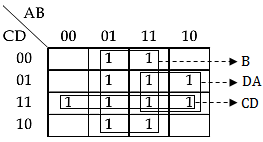Question 49
Postorder traversal of a given binary search tree T produces following sequence of keys:3, 5, 7, 9, 4, 17, 16, 20, 18, 15, 14 Which one of the following sequences of keys can be the result of an in-order traversal of the tree T?
 A 3, 4, 5, 7, 9, 14, 20, 18, 17, 16, 15 B 20, 18, 17, 16, 15, 14, 3, 4, 5, 7, 9 C 20, 18, 17, 16, 15, 14, 9, 7, 5, 4, 3 D 3, 4, 5, 7, 9, 14, 15, 16, 17, 18, 20
Algorithms       Searching
Question 49 Explanation:
We can easily find solutions based on given options because option D given all numbers in ascending order. In Order will print ascending order only.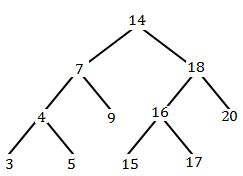Question 50
Which of the following statements is/are TRUE ?
(i) The grammar S → SS | a is ambiguous (where S is the start symbol).
(ii) The grammar S → 0S1 | 01S | e is ambiguous (the special symbol e represents the empty string and S is the start symbol).
(iii) The grammar (where S is the start symbol).
S → T/U
T → x S y ? xy ? e
U → yT
generates a language consisting of the string yxxyy.
 A Only (i) and (ii) are TRUE B Only (i) and (iii) are TRUE C Only (ii) and (iii) are TRUE D All of (i), (ii) and (iii) are TRUE
Theory-of-Computation       Context-Free-Language
Question 50 Explanation:
Option (i) parse tree is ambiguous.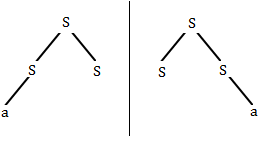Option (ii): For producing string 01 it gives 2 parse tree. So, it is also ambiguous.
Option (iii): It is also trueThere are 50 questions to complete.
PHP Code Snippets Powered By : XYZScripts.com
error: Content is protected !!